# Array Pointers in C Programming

Arrays are the list of values of same datatype stored in contiguous memory locations. They are accessed using the subscripts (0, 1, 2 etc) to the array name. We can notice the same in below array, intX of 3 elements.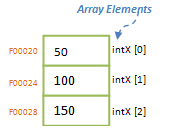But we have seen in pointer arithmetic that incrementing the pointer variable by one will point the pointer to next subsequent memory address. That means, if we increment integer pointer by one, then it will increment the address by 4 bytes, which is the address of next variable or in arrays it is the address of next element. This concept of pointer arithmetic can be used to have pointer to arrays. A pointer variable is created and made to point to an array. Initially, the pointer will be pointing to the beginning element of the array. As we increment the pointer variable, it goes on pointing to the subsequent element of the array. This type of pointer is called pointer to an array.

```#include <stdio.h>
int main()
{
int   intX[] = { 50, 100, 150 };
int   *intPtr;
int i;

intPtr = &intX; // assigns initial address of array intX to the pointer, i.e.; intPtr = F00020

for (i = 0; i <= 2; i++){
printf("Element of the array intX[%d] has value %d and is at address %x \n", i, *intPtr, intPtr);
intPtr++; // increments the pointer variable to point to next array element
}
return 0;
}```

In above program, pointer intPtr is made to point to the beginning of the array intX. Hence initially intPtr will have address F00020, which is the address of intX . As we increment the pointer by using intPtr++, it increments the address by 4 bytes every time and moves the pointer to subsequent elements of the array, as shown in below diagram. Please note that intPtr++ and intPtr+1 are same. Here pointer intPtr is called pointer to an array of 3 elements.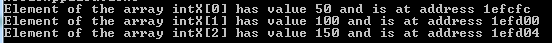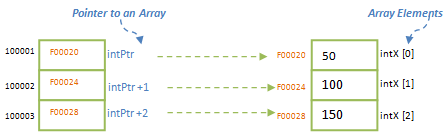When we simply write array name in the code, then it will always point to the beginning of the array, i.e.; first element in the array. Hence we can assign the address of array to the pointer variable by writing as below:

`intPtr = intX; // it is same as intPtr = &intX ;`

Please note that no ‘&’ or ‘*’ are used. Why? This is because, when array name is written without any index, it always point to the beginning of the array – points to first element of the array; but not the element. That means it points to the first element address. This implies that array also has some features of pointers! When array is written without index, it acts like a pointer. Hence if we have to access the first element of array using its pointer feature, we can write it as *(intX) which is same as intX . Next element can be accesses as *(intX+1), i.e.; intX . That means ith element in the array, intX [i] can also be accessed using *(intX +i). This is simple arithmetic of array address and the index. We know that arithmetic additions are commutative. Hence ith element can also be accessed as *(i+intX). From the initial steps of array’s pointer feature, *(i+intX) can also be written as i [intX]. That means array name can be index and index can be written as name!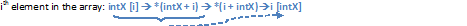Above rule signifies that if array can be written as intX [i], then it leads to same value when written as i [intX].

In above case we have created a simple pointer variable to point to an array. Can we have an array of pointers where array itself is created as pointers? Yes, we can have array of pointers where each element of the array is a pointer, i.e.; each element in the array has the address of the elements of another array. This concept of pointer array is different from pointer to an array discussed above. It may look same at the beginning but they are different concepts.

Suppose intX is an array of 3 elements and intPtr is an array of pointers. Note the difference between the declaration of array of pointers below (*intPtr []) and pointer to an array above (*intPtr). We can observe below that pointer itself is an array and its elements are the addresses of elements of intX. The code intPtr[i] = &intX[i] assigns the address of each elements to the array of pointers. Here we can access the elements of array using the pointer in the same way as we access the array. Only difference that we can note here is that with array of pointers, we need to use ‘*’ to access the value in the array. If we use intPtr[i], then it will give the address of ith element in the array.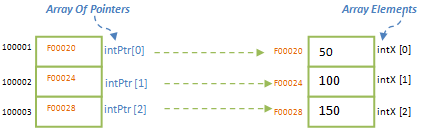```#include <stdio.h>
int main()
{
int   intX[] = { 50, 100, 150 };
int   *intPtr;
int i;

for (i = 0; i <= 2; i++) {
intPtr[i] = &intX[i]; // assigns address of each element of array, intX to array of pointers, intPtr []
}

for (i = 0; i <= 2; i++){
printf("Element of the array intX[%d] has value %d and is at address %x \n", i, *intPtr[i], intPtr[i]);
}
return 0;
}```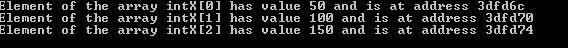Translate »